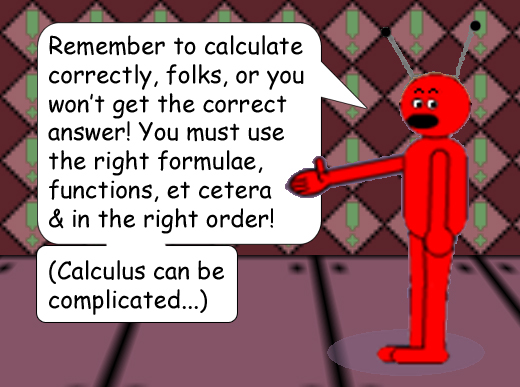# Complex Number Kookiness!

## This function says: "A complex number's absolute value to the power of any number is equal to the absolute value of the same complex number to the power of the same number!"

Note: The variable c must be a real number.

## Examples:

(3 + 4i)2 = -7 + 24i

|3 + 4i|2 = |-7 + 24i|

|3 + 4i| = 5; |-7 + 24i| = 25

[And 52 = 25]

### When you square complex numbers or multiply 2 different complex numbers, you must use what is called the F.O.I.L. rule: First 2 to the left inside the parentheses, Outer, Inner, Last 2 to the right inside the parentheses.(3 + 4i)3 = -117 + 44i

|3 + 4i|3 = |-117 + 44i|

|3 + 4i| = 5; |-117 + 44i| = 125

[And 53 = 125]

1 more...and I'll change the 1st complex number this time!

(8 + 7i)4 = -12,319 + 3,360i

|8 + 7i|4 = |-12,319 + 3,360i|

|8 + 7i| = the square root of 113; |-12,319 + 3,360i| = 12,769

[And the 4th root of 12,769 = the square root of 113 because 1132 = 12,769]